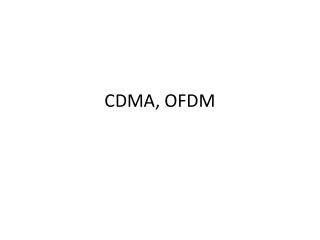DownloadDownload PresentationCDMA, OFDM

# CDMA, OFDM

Télécharger la présentation## CDMA, OFDM

- - - - - - - - - - - - - - - - - - - - - - - - - - - E N D - - - - - - - - - - - - - - - - - - - - - - - - - - -
##### Presentation Transcript

1. CDMA, OFDM

2. CDMA • Code Division Multiplexing

3. CDMA • Used in 3G networks. • Direct Sequence Spread Spectrum: spread a data bit into multiple chips. • Each sender has a unique chip sequence, that is *orthogonal* with other chip sequences.

4. Simple Examples of CMDA • A: (-1 -1 -1 +1 +1 -1 +1 +1 ) • B: (-1 -1 +1 -1 +1 +1 +1 -1 ) • C: (-1 +1 -1 +1 +1 +1 -1 -1 ) • D: (-1 +1 -1 -1 -1 -1 +1 -1 )

5. Orthogonal Frequency-Division Multiplexing • From the highest level, OFDM divides the communication bandwidth into a number of sub-channels, each occupying a fraction of the bandwidth. • Each sub-carrier is modulated by BPSK, QPSK, or other schemes. • Used in 802.11a and 802.11g.

6. OFDM • Why OFDM? • More resilient to multi-path fading. • Fast Fourier Transform (FFT) algorithm makes the computation efficient.

7. OFDM • How OFDM is implemented (following Tse & Viswanath book Ch. 3.4.4). • Assume the received sample is • hl is the channel coefficient at tap l. This is caused by multi-path – as we discussed earlier, a weak path will have a different delay compared to the main path. When we are taking sample at time m, the response excited by other data symbols will not be 0 at this point. As a result, the sample voltage contains some fraction from data symbols other than the symbol at time m.

8. OFDM • In this explanation, it is assumed that hl=0 for l <0 and l>=L. • Basically, we need to assume that the channel coefficients are not all nonzero, which it quite reasonable – the delay spread is usually bounded, and the impulse response is fast approaching 0 after several symbol time.

9. OFDM • Suppose we need to send Nc data symbols denoted as d to d[Nc-1]. • With OFDM, we not only send these data symbols, but also append a prefix of length L to it at the beginning. That is, we send d[Nc-L+1] , d[Nc-L+2] ,…, d[Nc-1] ,d, d,….,d[Nc-1]. • With these data symbols as input, the output at time m for L<=m<=Nc+L-1 is • Cyclic convolution. y[m] is the cyclic convolution of h and d.

10. OFDM • Given two series h and d, both are non-zero only within [0,N-1], the cyclic convolution of h and d is defined as • Cyclic convolution. Let the DFT of h[m], d[m] and y[m] be H[k],D[k] and Y[k],respectively. We have Y[k]=H[k]D[k].

11. OFDM • Cyclic convolution. If there is n’ such that for all m-n, Note that this is true if n’ = (m-n) mod N.

12. OFDM • The implication of this is that we may transmit the data symbols in the frequency domain. That is, • the data symbols are actually D[k], 0<=k<Nc. • Then we do a IDFT on D[k] to get d[n], 0<=n<Nc. • Then we append the cyclic prefix, and send out the block, called an OFDM block or an OFDM symbol. • The OFDM symbol will go through multipath, say we received y, y,…, y[L-1], y[L],…, y[Nc+L-1]. • We then take only y[L-1], y[L],…, y[Nc+L-1] and do a DFT on them. • Because y[L-1], y[L],…, y[Nc+L-1] is the cyclic convolution of d[n] and hl, the result of DFT is D[k]H[K]!

13. OFDM